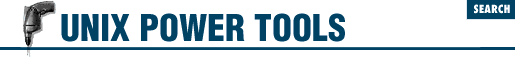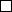home | O'Reilly's CD bookshelfs | FreeBSD | Linux | Cisco | Cisco Exam

#Chapter 30vi Tips and Tricks## 30.15 Useful Global Commands (with Pattern Matches)

The best way to learn pattern matching is by example, so here's a short list of pattern-matching examples, with explanations. (Article 26.10 has a list of these patterns.) Study the syntax carefully so you understand the principles at work. You should then be able to adapt these examples to your own situation.

1. Change all occurrences of the word help (or Help ) to HELP :

 ``` % ``` ``` ``` :%s/[Hh]elp/HELP/g ``` ```

or:

``````

:%s/[Hh]elp/\U&/g
```
```

The ``` \U``` changes the pattern that follows to all uppercase. The pattern that follows is the repeated search pattern, which is either help or Help .

2. Replace one or more spaces following a colon (``` :``` ) or a period (``` .``` ) with two spaces (here a space is marked by a \h'1p'\h'1p'):

``````

:%s/\([:.]\\)\h'1p'\h'1p'\h'1p'\h'1p'*/\\1\h'1p'\h'1p'\h'1p'\h'1p'/g
```
```

Either of the two characters within brackets can be matched. This character is saved into a hold buffer, using ``` \(``` and ``` \)``` ( 34.10 ) , and restored on the right-hand side by the ``` \1``` . Note that within brackets a special character such as a dot does not need to be escaped with a backslash (``` \``` ).

3. Delete all blank lines:

 ``` g ``` ``` ``` :g/^\$/d ``` ```

What you are actually matching here is the beginning of the line (``` ^``` ) followed by the end of the line (``` \$``` ), with nothing in between.

4. Delete all blank lines, plus any lines that contain only white space:

``````

:g/^[```
```

tab
```
```

]*\$/d
```
```

(In the line above, a tab is shown as ``` tab ``` .) A line may appear to be blank but may in fact contain spaces or tabs. The previous example will not delete such a line. This example, like the one above it, searches for the beginning and end of the line. But instead of having nothing in between, the pattern tries to find any number of spaces or tabs. If no spaces or tabs are matched, the line is blank. To delete lines that contain white space but that aren't blank, you would have to match lines with at least one space or tab:

``````

:g/^[```
```

tab
```
```

][```
```

tab
```
```

]*\$/d
```
```

5. Match the first quoted argument of all section header (``` .Ah``` ) macros ( 43.13 ) and replace each line with this argument:

``````

:%s/^\.Ah "\([^"]*\)" .*/\1/
```
```

The substitution assumes that the ``` .Ah``` macro can have more than one argument surrounded by quotes. You want to match everything between quotes, but only up to the first closing quote. Using ``` ".*"``` would be wrong because it would match all arguments on the line. What you do is match a series of characters that aren't quotes, ``` [^"]*``` . The pattern ``` "[^"]*"``` matches a quote, followed by any number of non-quote characters, followed by a quote. Enclose the first argument in ``` \(``` and ``` \)``` so that it can be replayed using ``` \1``` .

6. Same as previous, except preserve the original lines by copying them:

``````

:g/^\.Ah/t\$ | s/\.Ah "\([^"]*\)" .*/\1/
```
```

In ex , the vertical bar (``` |``` ) is a command separator that works like a semicolon (``` ;``` ) ( 8.5 ) on a UNIX command line. The first part, ``` :g/^\.Ah/t\$``` , matches all lines that begin with a ``` .Ah``` macro, uses the ``` t``` command to copy these lines, and places them after the last line (``` \$``` ) of the file. The second part is the same as in the previous example, except that the substitutions are performed on copies at the end of the file. The original lines are unchanged.

- TOR , DG from O'Reilly & Associates' Learning the vi Editor , Chapter 630.14 Moving Blocks of Text by Patterns30.16 Counting Occurrences; Stopping Search Wraps